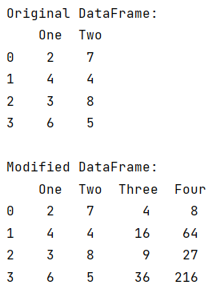# Pandas: Create two new columns in a DataFrame with values calculated from a pre-existing column

Given a Pandas DataFrame, we have to create two new columns in it with values calculated from a pre-existing column. By Pranit Sharma Last updated : September 24, 2023

Pandas is a special tool that allows us to perform complex manipulations of data effectively and efficiently. Inside pandas, we mostly deal with a dataset in the form of DataFrame. DataFrames are 2-dimensional data structures in pandas. DataFrames consist of rows, columns, and data.

## Creating two new columns in a DataFrame with values calculated from a pre-existing column

For this purpose, we will first define a function to apply certain operations and conditions and finally, we will create a new column with the values returned by the function.

Let us understand with the help of an example,

## Python program to create two new columns in a DataFrame with values calculated from a pre-existing column

```# Importing pandas package
import pandas as pd

# Defining a function
def function(x):
return x**2, x**3

# Creating a dictionary
d= {'One':[2,4,3,6],'Two':[7,4,8,5]}

# Creating a DataFrame
df = pd.DataFrame(d)

# Display original DataFrame
print("Original DataFrame:\n",df,"\n")

# Creating two new columns
df['Three'],df['Four'] = zip(*df['One'].map(function))

# Display modified DataFrame
print("Modified DataFrame:\n",df)
```

### Output

The output of the above program is: